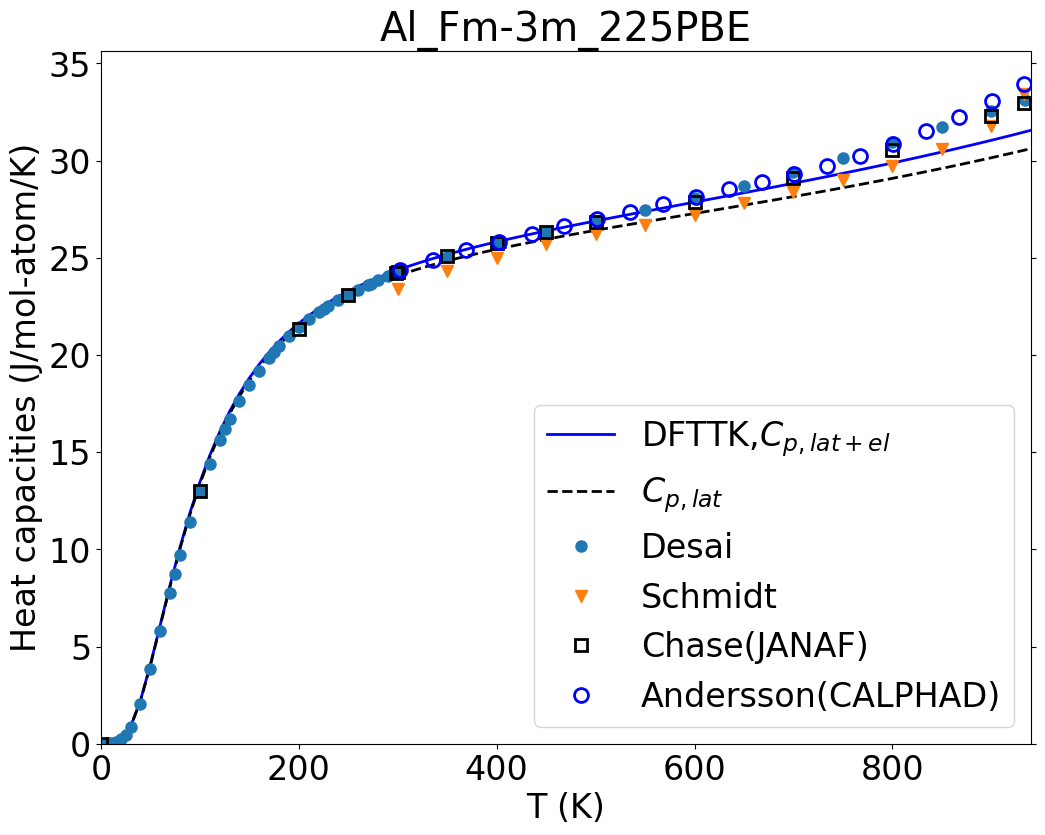#Welcome to dfttk_example’s documentation!¶

## Example¶

To get the example, run

```git clone https://github.com/yiwang62/dfttk_example #if you have not download the example
cd dfttk_example
```

The example is designed to have the user to test the DFTTK package using Al. The input settings for the Al example are contained in the `Al/` folder by two files

• `POSCAR` - the regular VASP POSSCAR file

• `SETTINGS.yaml` - the setting file for quasiharmonic phonon calculation

The following gives the steps to run the `Al` example

```cd Al
dfttk run -wf robust -f POSCAR -l -m 1
```

This will submit the batch DFT job to the system. One can check the progress of the DFT calculations by `lpad get_wflows`. Only when all the values for the ‘states_list’ fields are shown as ‘C’ implies the DFT job done. Then One can go to next step by run

```cd dfttk_example #go back the dfttk example folder
dfttk thfind -get -plot DFTTK -expt ExptData.json
```

The file `ExptData.json` under the dfttk_example folder contains some experimental thermodynamic data for a collection of materials to verify the DFTTK calculations. The above will produce more thatn 20 figures stored in the folder `Al_Fm-3m_225PBE/figures` and they can be viewed t by clicking them in Windows/IOS or using the linux command `display` to show the figure. For example for linux

```display Al_Fm-3m_225PBE/figures/LTC.png #to see the linear thermal expansion coefficient
``````display Al_Fm-3m_225PBE/figures/Heat_capacities.png #to see the heat capaticity, and so on
```The `Al_Fm-3m_225PBE/` folder contains all calculated thermodynamic properties after post-processing the data stored in the MongoDB database for a finished DFT calculations, in particular

• `figures/` - plots in png format for most of the thermodynamic properities

• `readme` - extensive summary of the calculated results in json format

• `fvib_ele` - tablated data containing the calculated thermodynamic properties

• `fvib_eij` - tablated data containing the calculated thermal expansion coefficient tensor

• `record.json` - SGTE fitting record for heat capacity, Gibbs energy, enthalpy, and entropy at given temperature range